Courses

# Extra Questions and Practice Exercise- Squares and Square Roots Class 8 Notes | EduRev

## Class 8 : Extra Questions and Practice Exercise- Squares and Square Roots Class 8 Notes | EduRev

The document Extra Questions and Practice Exercise- Squares and Square Roots Class 8 Notes | EduRev is a part of the Class 8 Course Class 8 Mathematics by VP Classes.
All you need of Class 8 at this link: Class 8

Question 1: How many numbers lie between squares of:

(i) 27 and 28 (ii) 16 and 17 (iii) 97 and 98

Solution: We know that, between n2 and (n + 1)2, there are 2n non-square numbers.

∴ (i) Between 27 and 28, there are 2 * 27, i.e 54 numbers.

(ii) Between 16 and 17, there are 2 * 16, i.e. 32 numbers.

(iii) Between 97 and 98, there are 2 * 97, i.e. 194 numbers.

Question 2: Express 169 as the sum of first 13 odd numbers.

Solution: We have 169 = 132

= Sum of first 13 odd numbers

= 1 + 3 + 5 + 7 + 9 + 11 + 13 + 15 + 17 + 19 + 21 + 23 + 25

= (1 + 25) + (3 + 23) + (5 + 21) + (7 + 19) + (9 + 17) + (11 + 15) + 13

= 26 + 26 + 26 + 26 + 26 + 26 + 13

= (6 * 26) + 13 = 156 + 13 = 169

Question 3: Write the Pythagorean triplet whose one member is 13.

Solution: When ‘n’ is a member of a Pythagorean triplet, then the triplet is

n2 – 1, 2n, n2 + 1

or (132 – 1), (2 * 13), (132 + 1)

or (169 – 1), (26), (169 + 1)

or 168, 26 and 170

Question 4: Using prime factors, find the square root of 729.

Solution: We have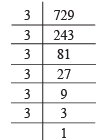∴ 729 = 3 * 3 * 3 * 3 * 3 * 3

= 32 * 32 * 32

∴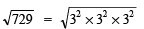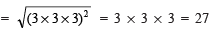Question 5: Find the smallest whole number by which 288 should be divided so as to get a perfect square. Also find the square root of the resulting number.

Solution: We have

288 = 2 * 2 * 2 * 2 * 2 * 3 * 3

= 22 * 22 * 2 * 32

= (2 * 2 * 3)2 * 2

⇒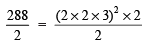⇒ 144 = (2 * 2 * 3)2

⇒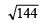= 2 * 2 * 3 = 12

Thus, 288 is to be divided by 2 to get a perfect square.

i.e. The required smallest number = 2

Also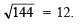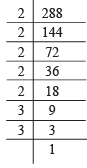Question 6: Find the length of the side of a square whose area is 676 m2.

Solution: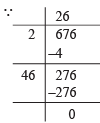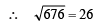Now, let the side of the square = x m

∴  Area = x2

⇒ x2 = 676

⇒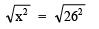⇒ x= 26

∴ The required side of the square = 26 m

Question 7: In a right triangle ABC, ∠ B = 90°. If AB = 12 cm, BC = 5 cm, then find AC.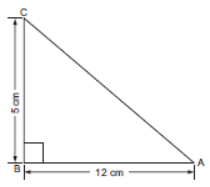Solution: We know that, in a right triangle, the side opposite to 90° is hypotenuse.

∴ AC is the hypotenuse in DABC.

According to Phythagoras theorem,

(Hypotenuse)2 = [Sum of the squares of the other two sides]

∴ AC2 = AB2 + BC2

⇒  AC2 = (12)2 + (5)2

⇒ AC2 = 144 + 25

⇒ AC2 = 169 = (13)2

⇒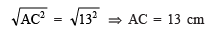Offer running on EduRev: Apply code STAYHOME200 to get INR 200 off on our premium plan EduRev Infinity!

93 docs|16 tests

,

,

,

,

,

,

,

,

,

,

,

,

,

,

,

,

,

,

,

,

,

;